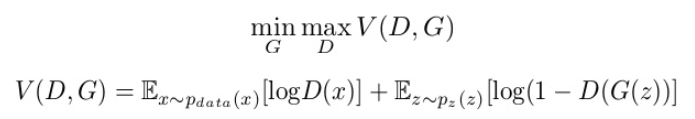# Query regarding the minmax loss function formulation of the training of a Generative Adversarial Network (GAN)

Just needed a clarification on the training procedure for a standard GAN. Of my understanding the loss function to optimize is a min max (max min causing mode collapse due to focus on one class generation) problem where the loss functionneeds to maximized for the discriminator and minimized for the generator networks -

1.) In this equation are the $$E_{z~p_{z(z)}}$$ and $$E_{x~p_{data(x)}}$$ the means of the distributions of the mini batch samples? Also is the optimal case for the discriminator a maximum value of 0? and the optimal case for the generator a minimum value of $$log(small~value)$$ {basically a massive negative value}? If so, what happens to the first term during the training of the generator - is it taken as a constant or is the discriminator performing badly considered optimal for the generator?

2.) While putting this in code, for every step is the discriminator trained first for one step, keeping the generator constant, followed by the generator being trained for the same step, with the discriminator kept constant?

3.) In every step of training are there multiple latent vectors sampled to produce multiple generator outputs for each step? If so is the loss function an average or sum of all $$V(D, G)$$ for that step

• Hi. Please, ask one question per post next time!! – nbro Jul 24 '19 at 8:02

In this equation are the $$E_{z \sim p_z(z)}$$ and $$E_{x \sim p_{data}(x)}$$ the means of the distributions of the mini batch samples?

So let's take the first part $$E_{x \sim p_{data}(x)}[log \,D(x)]$$. This is read as the "expected value of $$log \, D(x)$$, where $$x$$ is sampled from $$p_{data}(x)$$". So, in simpler terms this means that:

• You have a distribution $$p_{data}(x)$$.
• You sample a batch of samples $$x$$ from this distribution.
• You feed the batch to the discriminator and get its output $$D(x)$$.
• You compute the log for the batch of predictions.
• You average over the samples in the batch.

Also is the optimal case for the discriminator a maximum value of 0?

Yes, the goal of the discriminator is to maximize $$V(D, G)$$. This is achieved when $$D(x) = 1$$ and $$D(z) = 0$$, where $$V(D, G)=0$$.

the optimal case for the generator a minimum value of log(small value) {basically a massive negative value}?

Yes, the generator wants to minimize $$V(D, G)$$, so it wants $$D(x)$$ and $$D(z)$$ to be as small as possible (though it can affect only the second term). The minimum value for $$D(z)$$ is $$1 / M$$, where $$M$$ is the number of classes in the dataset. So the minimum value for $$V(D, G)$$ is $$\log{[(M-1) / M^2]}$$ (I think).

If so, what happens to the first term during the training of the generator - is it taken as a constant or is the discriminator performing badly considered optimal for the generator?

The first term is of no consequence to the Generator, because the generator can't affect it somehow (i.e. like you say it is taken as a constant). Consider the generator $$G_{\theta}$$ has parameters $$\theta$$ and the discriminator $$D_{\phi}$$ has parameters $$\phi$$. When training $$G$$:

$$\frac{\log{D_{\phi}(x)}}{\partial \theta} = 0$$

So the generator is trained of $$V$$.

While putting this in code, for every step is the discriminator trained first for one step, keeping the generator constant, followed by the generator being trained for the same step, with the discriminator kept constant?

In theory, yes. In practice for every step the Generator takes, we train the Discriminator for more steps (e.g. $$5$$ steps).

In every step of training are there multiple latent vectors sampled to produce multiple generator outputs for each step? If so is the loss function an average or sum of all V(D,G) for that step.?

Yes, at every step we sample a batch of latent vectors. The equation indicates that we average over these (i.e. the expectation) but in practice it doesn't have any difference (the average is the sum divided by a constant number, which doesn't affect the optimization very much).